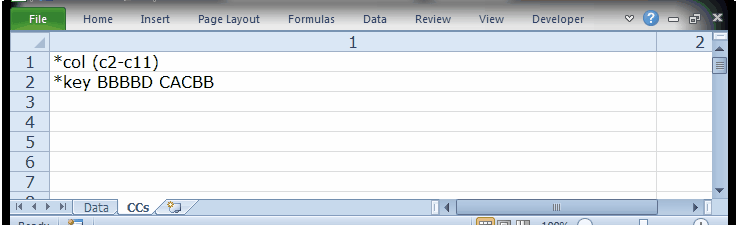﻿ ChemQuiz

# Example 1: "ChemQuiz", a short cognitive test

A high school chemistry teacher developed a short quiz based the periodic table.

The quiz consisted of 10 multiple-choice questions. Each question had four options: A, B, C, and D.

The teacher gave the quiz to his class, and recorded the responses of each student in an Excel worksheet called "Data". These responses are captured here:Four students did not answer some of the questions. For example, for some reason Klein did not answer two items, Q2 and Q7.

There is much information which the teacher could glean from the Data worksheet itself. There were so few students; he could, without much trouble, rather quickly summarise how many students got each item correct, and which students selected one of the "distractors" (wrong answers).

However, we want to show you what Lertap does, so let's move on, imagining the day when there will be many students.

Lertap requires two Excel worksheets before it'll do anything. Item responses have to be in a Data worksheet, with the first row used for a concise summary of the information in the worksheet, the second row for column headers, and then the actual student responses, starting in row 3.

We also have to have a "CCs" worksheet with instructions for Excel. The teacher knew this, and he entered these two lines of information in the syntax common to Lertap:The purpose of the first CCs line, *col, is to tell Excel that item responses are found in columns 2 through 11 of the Data worksheet. The second CCs line, *key, gives Excel the correct answer to each question; these are referred to as "keys". There are 10 keys in the line, one for each of the 10 questions. The correct answer to the first item, Q1, is B. The correct answer, or the "key", for the 7th item is A.

(Note: the space between the 5th and the 6th key is not required. Grouping keys in sets of 5 makes it easier to quickly identify, for example, the keyed-correct answer to the seventh item, or the ninth, and so on. There's also a space before the first key, as there is before the opening parenthesis on the *col line; these spaces are required.)

People sometimes ask what "CCs" stands for. The answer is: "Control Cards". The term dates back to the mainframe version of Lertap, to a time when "punch cards" were used to enter data, and to specify how a batch of punched cards was to be processed by the computer. (For more information about punch  cards, search the internet.)

The next topic shows how Lertap can be used to process the students' responses.